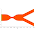# 2-D, steady, Kovasznay flow

In this section, the exact and numerical solutions of the Kovasznay flow in vorticity-stream function formulation is presented. The closed-form solution of 2-Dimensional steady Kovasznay flow reads
\begin{equation} \Psi = y - \frac{e^{\lambda x}}{2\pi}\sin 2\pi y, \end{equation} \begin{equation} \Omega = \frac{\lambda^2-4\pi^2}{2\pi}e^{\lambda x}\sin 2\pi y, \end{equation} where $\lambda = (\mbox{Re}/2) - \sqrt{4\pi^2+(\mbox{Re}^2/4)}$.
This exact solution of the Navier-Stokes equations can be employed to verify the numerical solvers. In order to solve the Kovasznay flow numerically, the following system of equations are discretized using second-order finite difference scheme
\begin{equation} \frac{\partial \Psi}{\partial y} \frac{\partial \Omega}{\partial x} - \frac{\partial \Psi}{\partial x} \frac{\partial \Omega}{\partial y} = \frac{1}{\mbox{Re}} \left(\frac{\partial^2 \Omega}{\partial x^2} + \frac{\partial^2 \Omega}{\partial y^2} \right), \end{equation} \begin{equation} \Omega = -\left(\frac{\partial^2 \Psi}{\partial x^2} + \frac{\partial^2 \Psi}{\partial y^2} \right). \end{equation}
The numerical results for the stream function, $\Psi$, and vorticity, $\Omega$, are shown in the following figures, respectively.

Fig. d.8: Streamlines and vorticity contours for $\mbox{Re} = 12$.

To show grid convergence, calculations were carried out for different grid sizes, from $40 \times 40$ up to $160 \times 160$. Fig. d.9 shows the infinity norm of the deviation of computed stream function from the exact solution as a function of the grid size, $N = \sqrt{N_x \times N_y}$. The slope of the curve confirms the second-order convergence rate of the numerical scheme.

Fig. d.9: Convergence study.

The relevant Matlab codes can be downloaded using the following link:
Matlab Codes Bank

Back to 1-D non-linear partial differential equations

#### 1 comment:

1.how i can do work in free fem? can some one help me?